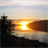QlikView App Dev

Discussion Board for collaboration related to QlikView App Development.

Announcements
QlikWorld 2022, LIVE in Denver CO., May 16-19, 2022. REGISTER NOW TO RECEIVE EARLY BIRD PRICING
cancel
Showing results for
Did you mean:Creator II

Set Analysis and max date

Hello,  I'm trying to compare amounts based on the max month available.

My issue occurs whenever I select a month, the expressions sum the selected month's amount instead of the previous month's amount.

Here's an example of my code

First Column:

=sum({<Reporting_Period_Num = {'=(max(Reporting_Period_Num))'}>} Account_Balance)

Second column:

=sum({<Reporting_Period_Num = {'=(max(Reporting_Period_Num)-1)'}>} Account_Balance)

1 Solution

Accepted SolutionsMVP

I think you will need to add other mastercalendar fields in here to work:

=Sum({<Reporting_Period_Num = {"\$(=Max(Reporting_Period_Num)-1)"}, Month = , Year = , Date = >} Account_Balance)

or try this with a 1 to restrict any selections except the one (Reporting_Period_Num)

=Sum({1<Reporting_Period_Num = {"\$(=Max(Reporting_Period_Num)-1)"}>} Account_Balance)

14 RepliesMVP

You mean your second column shows you the sum for the selected period?Creator II
Author

Both columns do.  When a month is not selected, it shows the sum for all periods as if the set analysis is being ignoredMVP

See if this helps:

First Column:

=Sum({<Reporting_Period_Num = {"\$(=Max(Reporting_Period_Num))"}>} Account_Balance)

Second column:

=Sum({<Reporting_Period_Num = {"\$(=Max(Reporting_Period_Num)-1)"}>} Account_Balance)Creator II
Author

That just gives me 0's across the boardMVP

I made some changes to the expression, did you use these?

First Column:

=Sum({<Reporting_Period_Num = {"\$(=Max(Reporting_Period_Num))"}>} Account_Balance)

Second column:

=Sum({<Reporting_Period_Num = {"\$(=Max(Reporting_Period_Num)-1)"}>} Account_Balance)

If not, try them. If you did, then can you share some date to check?Creator II
Author

Close.  It works now until I select a month, then the column that takes the max period-1 turns to 0MVP

I think you will need to add other mastercalendar fields in here to work:

=Sum({<Reporting_Period_Num = {"\$(=Max(Reporting_Period_Num)-1)"}, Month = , Year = , Date = >} Account_Balance)

or try this with a 1 to restrict any selections except the one (Reporting_Period_Num)

=Sum({1<Reporting_Period_Num = {"\$(=Max(Reporting_Period_Num)-1)"}>} Account_Balance)Creator II
Author

That works!  The 1 in front is making the difference.  Thank you so much!!MVP

But make sure you understand what it is doing. 1 in the front will make the expression not respond to any selection except Reporting_Period_Num. Is that what you want? If not, I would suggest using the other expression with all the master calendar fields in it.

Best,

SunnyCommunity Browser